# RD Sharma Solutions for Class 6 Maths Chapter 22: Data Handling - II (Pictographs) Exercise 22.2

Pictographs are very popularly used in representation of statistical data. Exercise 22.2 has problems which help in constructing pictographs for the given questions. The illustrative examples before the exercise help students understand the steps to be followed in solving problems. The solutions PDF created by subject matter experts help students to gain speed in solving RD Sharma textbook problems effortlessly. The students can avail free RD Sharma Solutions Class 6 Maths Chapter 22 Data Handling – II (Pictographs) Exercise 22.2 PDF from the links which are provided here.

## RD Sharma Solutions for Class 6 Maths Chapter 22: Data Handling – II (Pictographs) Exercise 22.2 Download PDF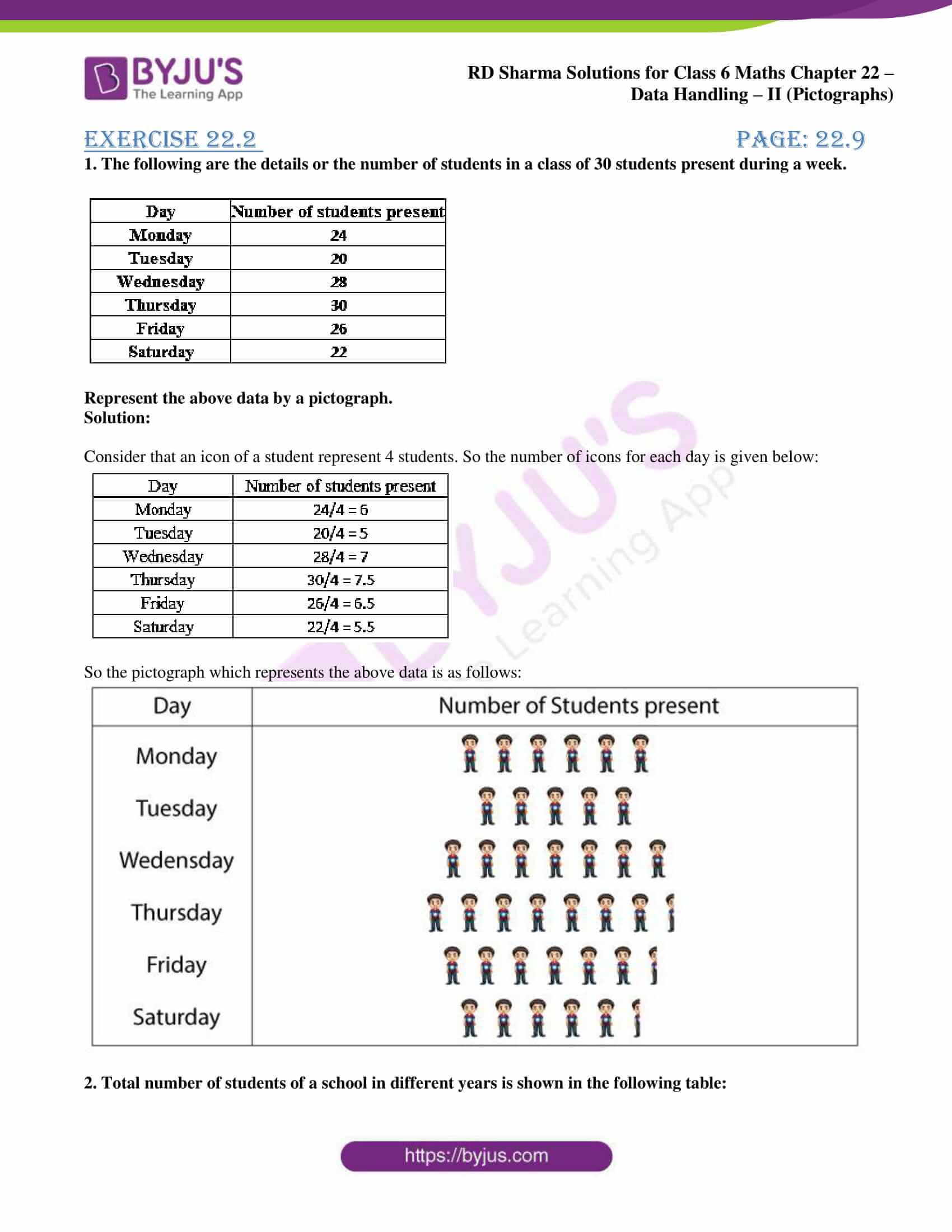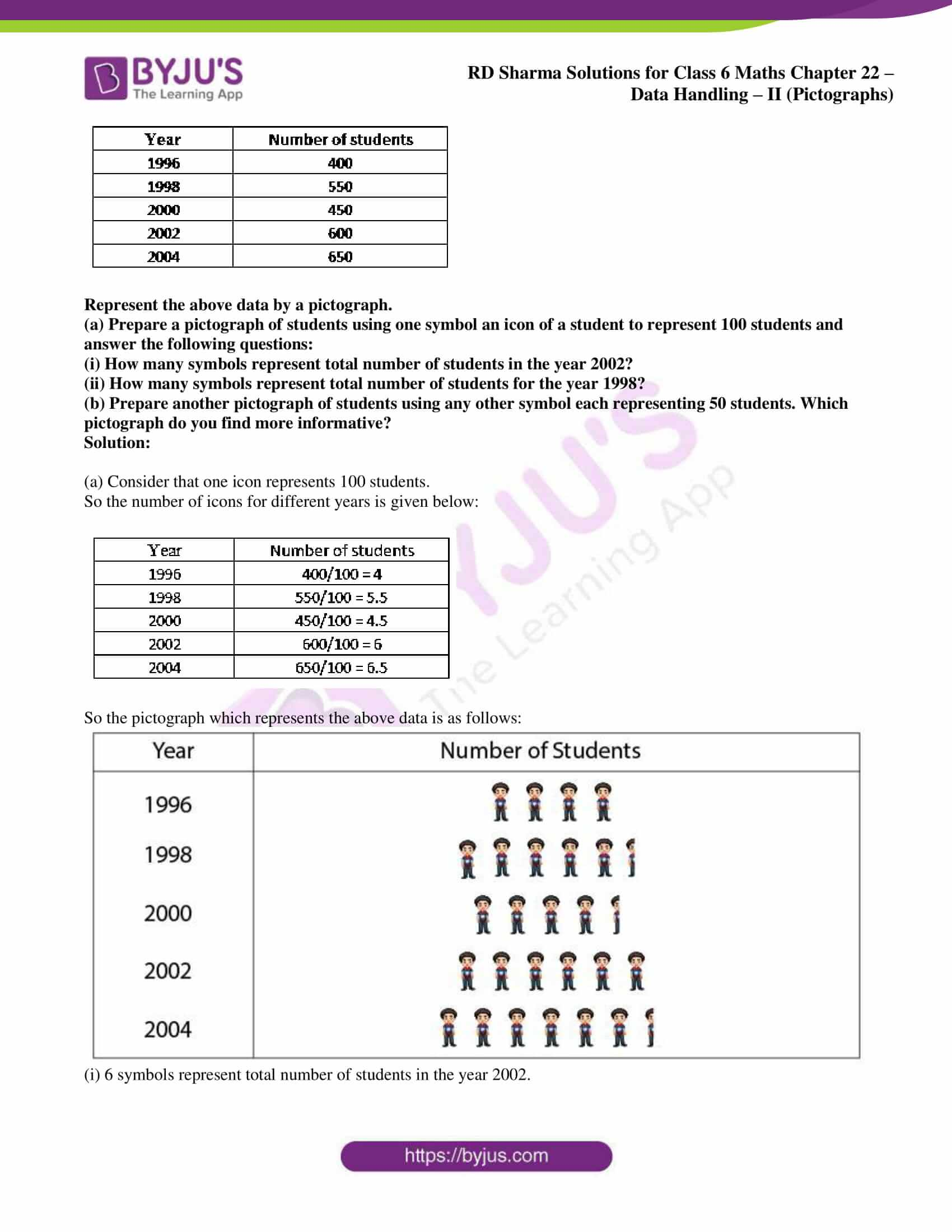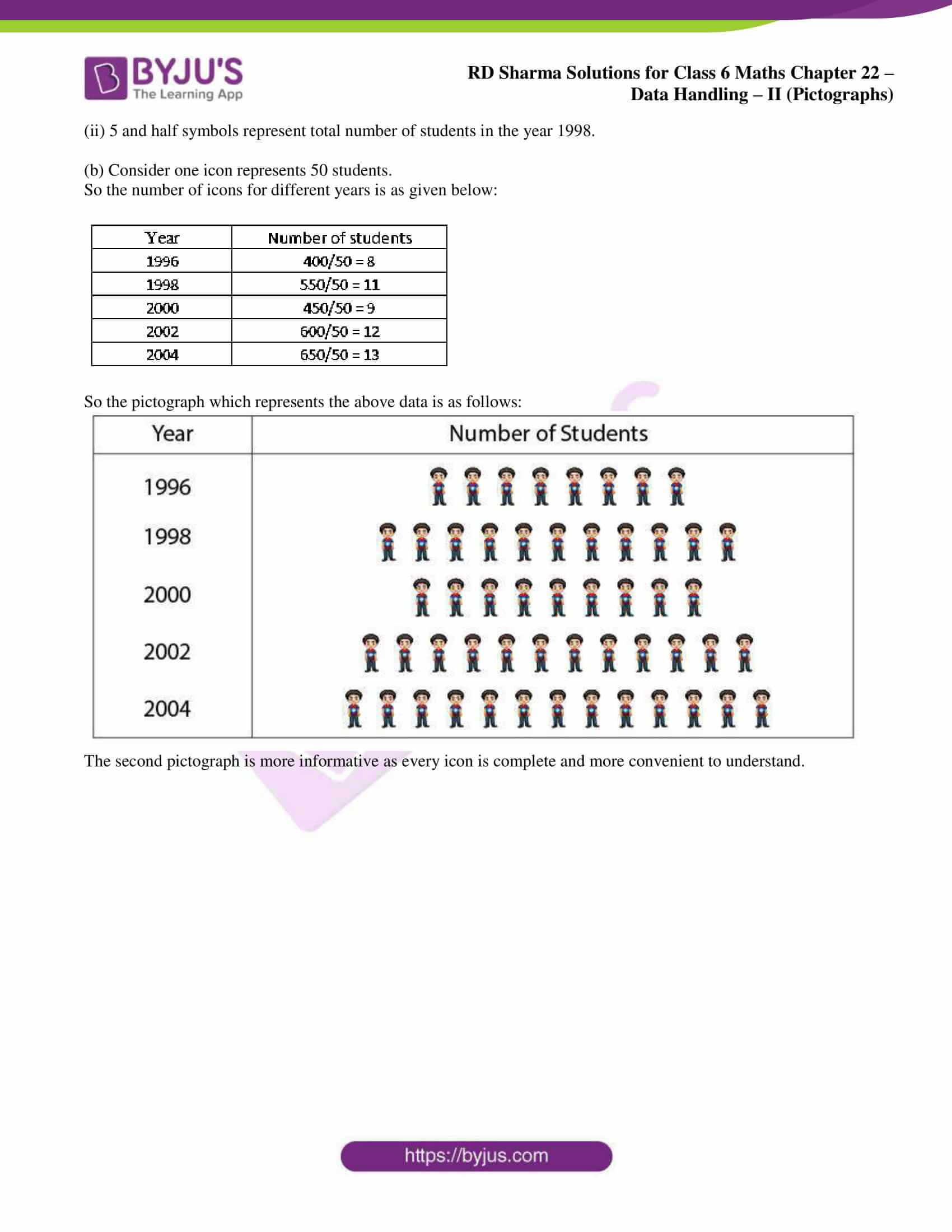### Exercise 22.2 page: 22.9

1. The following are the details or the number of students in a class of 30 students present during a week.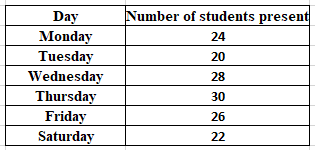Represent the above data by a pictograph.

Solution:

Consider that an icon of a student represent 4 students. So the number of icons for each day is given below: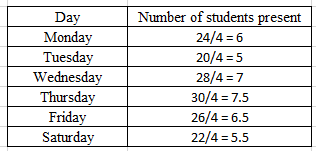So the pictograph which represents the above data is as follows: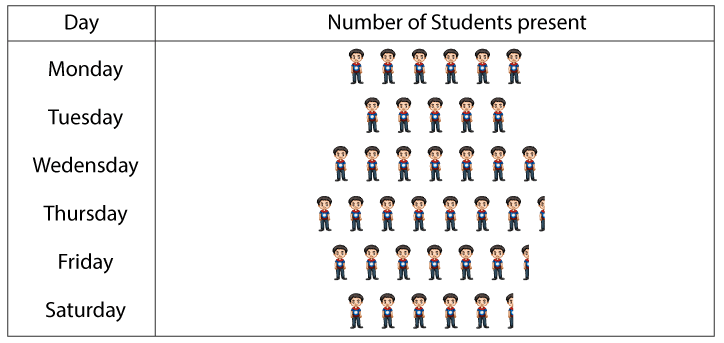2. Total number of students of a school in different years is shown in the following table: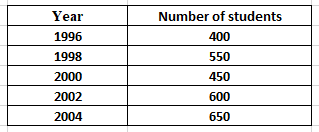Represent the above data by a pictograph.

(a) Prepare a pictograph of students using one symbol an icon of a student to represent 100 students and answer the following questions:

(i) How many symbols represent total number of students in the year 2002?

(ii) How many symbols represent total number of students for the year 1998?

(b) Prepare another pictograph of students using any other symbol each representing 50 students. Which pictograph do you find more informative?

Solution:

(a) Consider that one icon represents 100 students.

So the number of icons for different years is given below: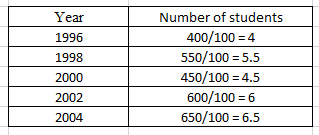So the pictograph which represents the above data is as follows: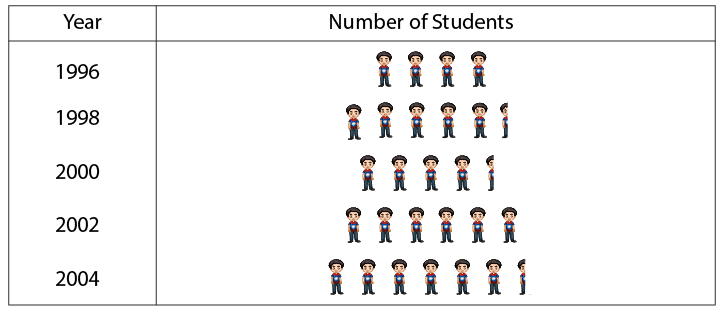(i) 6 symbols represent total number of students in the year 2002.

(ii) 5 and half symbols represent total number of students in the year 1998.

(b) Consider one icon represents 50 students.

So the number of icons for different years is as given below: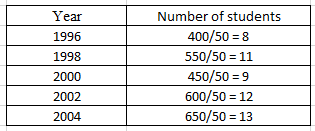So the pictograph which represents the above data is as follows: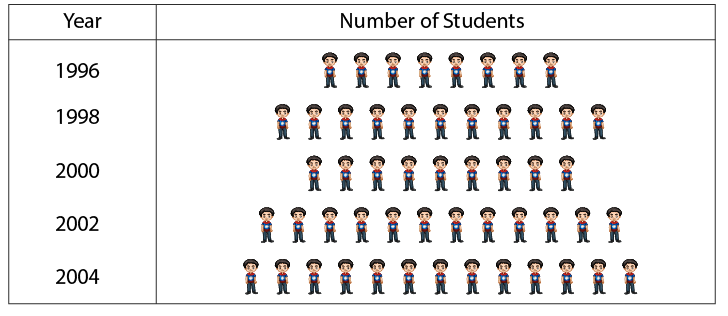The second pictograph is more informative as every icon is complete and more convenient to understand.